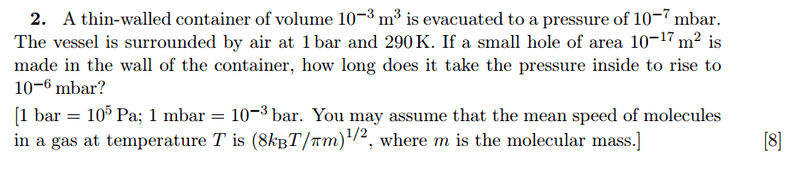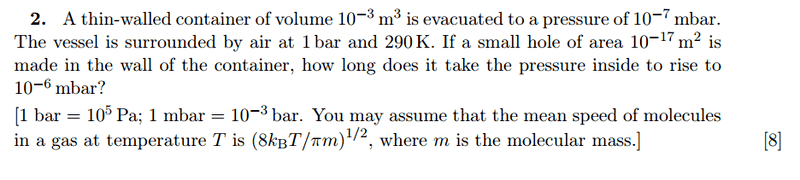# Effusion of molecules into a Box through a hole

## Homework Statement

A container of volume 10−3 m3 has a pressure of 10−7 mbar.
Surrounded by air at 1 bar and 290 K, how long does it take the pressure inside to rise to 10−6 mbar when air effuses through a small hole of area 10−17 m2?## The Attempt at a Solution

$$Flux ø = \frac{1}{4}n<v>$$

Starting from continuity equation:

$$øA = -\frac{dN}{dt}$$
$$\frac {1}{4} \frac{NA}{V}<v> = -\frac{dN}{dt}$$
$$n = n_0e^-{\frac{1}{4} \frac {A<v>t}{V} = n_0 e^-{bt} , b = {\frac{1}{4} \frac {A<v>}{V}$$

Am I right to assume that temperature in the box is the same as temperature of the surrounding air, 290K? Since average temperature of air molecules entering the box is 290K, the average temperature in the box is also 290K, which is constant.

$$\frac {P_f}{P_i} = \frac {n_f}{n_i} = e^{-bt}$$

Solving, $$t = 1.94 * 10^{12} s$$ The result is astronomical!

1. Homework Statement [/b]

Problem with the 4th equation , can't edit it, so i'm reposting it!

A container of volume 10−3 m3 has a pressure of 10−7 mbar.
Surrounded by air at 1 bar and 290 K, how long does it take the pressure inside to rise to 10−6 mbar when air effuses through a small hole of area 10−17 m2?## The Attempt at a Solution

$$Flux ø = \frac{1}{4}n<v>$$

Starting from continuity equation:

$$øA = -\frac{dN}{dt}$$
$$\frac {1}{4} \frac{NA}{V}<v> = -\frac{dN}{dt}$$
$$n = n_0 e^{-\frac{1}{4} \frac {A<v>t}{V}} = n_0 e^{-bt}$$,
$$b = {\frac{1}{4} \frac {A<v>}{V}}$$

Am I right to assume that temperature in the box is the same as temperature of the surrounding air, 290K? Since average temperature of air molecules entering the box is 290K, the average temperature in the box is also 290K, which is constant.

$$\frac {P_f}{P_i} = \frac {n_f}{n_i} = e^{-bt}$$

Solving, $$t = 1.94 * 10^{12} s$$ The result is astronomical!

DrClaude
Mentor
Can't you just use the flux of molecules coming into the vessel through the hole while neglecting molecules coming out (due to the huge difference in pressure)?

Can't you just use the flux of molecules coming into the vessel through the hole while neglecting molecules coming out (due to the huge difference in pressure)?

The flux that's entering the hole is ##\frac{1}{4}n<v>##. Solving the differential equation tells us its exponential. But I do not know the volume of the surroundings to solve for the constant b!

Can't you just use the flux of molecules coming into the vessel through the hole while neglecting molecules coming out (due to the huge difference in pressure)?

Flux into the container ø = ##\frac{1}{4}n_{air}<v>##
$$\frac{N}{A} = \frac{1}{4} \frac {P_{air}}{kT_{air}} \sqrt{\frac{8kT}{\pi m}}$$

I assume RHS = constant, since the surrounding air is huge, and ##n_{air}## shouldn't change much.

$$N = A* \frac{1}{4} \frac {P_{air}}{kT_{air}} \sqrt{\frac{8kT}{\pi m}} * t$$

$$\frac{PV}{kT} = A* \frac{1}{4} \frac {P_{air}}{kT_{air}} \sqrt{\frac{8kT}{\pi m}} * t$$

Subsituting ##P = 10^{-4}##,## V = 10^{-3}##, I still lack the temperature of the gas in the vessel..

DrClaude
Mentor
I would assume that temperature to be the same as that of the air. In a sense, it depends on how you read between the lines of the problem, which is influence by the level of the course.

If this is basic thermodynamics, then I would take this as calculating how much time the hole would have to be left open, such that after it has been closed and the system has had time to equilibriate with the sourroundings the pressure gauge would read 10-6 mbar. There are many assumptions that can be made there to trasnform this into a simple problem.

If this is a more advanced course, then it could mean that you have to take into account the expansion of the gas coming into the vessel, and figure out when a gauge would read that pressure (if the gauge gave instantaneous results). Then you have to contend with the fact that the walls are diabatic, and it gets much more complicated.

My assumption from the beginning was that the first case applies, and that you should try to find a simple representation of the problem. And since the problem mentions thin walls, I guess you should assume that the temperature in the vessel is the same as that of the surrounding air.

•1 person
I would assume that temperature to be the same as that of the air. In a sense, it depends on how you read between the lines of the problem, which is influence by the level of the course.

If this is basic thermodynamics, then I would take this as calculating how much time the hole would have to be left open, such that after it has been closed and the system has had time to equilibriate with the sourroundings the pressure gauge would read 10-6 mbar. There are many assumptions that can be made there to trasnform this into a simple problem.

If this is a more advanced course, then it could mean that you have to take into account the expansion of the gas coming into the vessel, and figure out when a gauge would read that pressure (if the gauge gave instantaneous results). Then you have to contend with the fact that the walls are diabatic, and it gets much more complicated.

My assumption from the beginning was that the first case applies, and that you should try to find a simple representation of the problem. And since the problem mentions thin walls, I guess you should assume that the temperature in the vessel is the same as that of the surrounding air.

Thanks. So it's okay to assume that the number density and temperature of surroundings in air doesn't change (since it's much more massive than the box)

DrClaude
Mentor
So it's okay to assume that the number density and temperature of surroundings in air doesn't change (since it's much more massive than the box)
Definitely.